Next: Development of Orientation Up: Associative Decorrelation Dynamics Previous: Dynamic Stability

## Optimal Information Transmission

Suppose that the output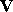of a network is the linear transformation of it inputplus some output noise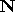, i.e.,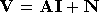, and both inputand noiseare Gaussian signals. Without losing generality, suppose that the network capacity is 1.

The optimal information transmition requires to choose the transformation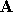which maximizes the information of the ouput about the input while keeping the output variance equal to the capacity. This can be easily solved by Lagrangian multiplier method through maximizing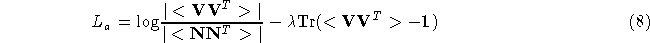in which the first term is the information of the output about the input and the second term is the Lagangian multiplier. The derivative of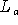at the maximum isThe solution is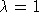and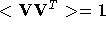, i.e., the output activities are decorrelated.

The dynamics of feedforward connections is very similar to the feedback case. We are not going into the detail of that since our emphasis is on the feedback path. The dynamic stability and information optimization still hold. It is not difficult to show that the functionsandshown earlier are still the Lyapunov function and Lagrangian function, respectively, for the joint dynamics of feedforward and feedback connections. The decorrelation of output activities is still the stable solution.Next: Development of Orientation Up: Associative Decorrelation Dynamics Previous: Dynamic Stability